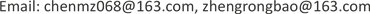1广东外语外贸大学，社会与公共管理学院，广东 广州

2广东工业大学，管理学院，广东 广州1. 引言

2. 研究区土地股份合作制概况

3. 收益分配主体演化博弈模型

3.1. 基本假设及参数假设

Return matrix of the government, economic association and village

D1 − C4 + C2 − C5−C4 + C3D1 − C4 − C5−C4
S1 − C1 − C2 + TT − C1 − C2−S1 − C20

C2 − C4 − C5C2 − C4−C4 − C5−C4
S1 + S2 − C1 − C2S2 − C1 − C2−S10

3.2. 演化博弈模型的建立

V G 1 = ( S 1 + T − C 1 − C 2 ) y + ( S 1 z + S 2 − C 1 − C 2 ) ( 1 − y ) (1)

V G 2 = − C 2 z y − S 1 z (2)

V G ¯ = V G 1 x + V G 2 ( 1 − x ) (3)

U ( x ) = d x d t = [ ( T − S 2 + C 2 ) y + S 2 − C 1 − C 2 ] x ( 1 − x ) (4)

U ( y ) = d y d t = [ ( S 2 − T ) x + ( R − D 1 ) z + I 1 − I 2 ] y ( 1 − y ) (5)

U ( z ) = d z d t = [ C 2 x + ( D 1 − C 3 + C 2 ) y − C 5 − C 2 + C 4 ] z ( 1 − z ) (6)

E 9 = [ 0 , − ( I 2 − I 1 ) ( T − S 2 ) − 1 , 0 ] ， E 10 = [ 1 , − ( I 2 − I 1 ) ( T − S 2 ) − 1 , 0 ] ， E 11 = [ 0 , − ( D − 1 R − I 1 + I 2 ) ( T − S 2 ) − 1 , 1 ] ，

E 12 = [ 1 , − ( D − 1 R − I 1 + I 2 ) ( T − S 2 ) − 1 , 1 ] ， E 13 = [ ( C 3 − C 4 + C 5 − D 1 ) C 2 − 1 , 1 , ( C 1 + C 2 − T ) C 2 − 1 ] ，

E 14 = [ 0 , − ( C 4 − C 2 − C 5 ) ( C 2 − C 3 + D 1 )

E 15 = [ 1 , − ( C 4 − C 5 ) ( C 2 − C 3 + D 1 ) − 1 , − ( I 1 − I 2 ) ( R − D 1 )

3.3. Jacobi稳定性分析

I = [ [ ( T − S 2 + C 2 ) y + S 2 − C 1 − C 2 ] ( 1 − 2 x ) ( T − S 2 + C 2 ) x ( 1 − x ) 0 ( S 2 − T ) y ( 1 − y ) [ ( S 2 − T ) x + ( R − D 1 ) z + I 1 − I 2 ] ( 1 − 2 y ) ( R − D 1 ) y ( 1 − y ) C 2 z ( 1 − z ) ( D 1 − C 3 + C 2 ) z ( 1 − z ) [ C 2 x + ( D 1 − C 3 + C 2 ) y − C 5 − C 2 + C 4 ] ( 1 − 2 z ) ]

I 1 = [ S 2 − C 1 − C 2 0 0 0 I 1 − I 2 0 0 0 − C 5 − C 2 + C 4 ]

Parameter assignmen

Characteristic root and stability analysis of Jacobi matri

E 1 = [ 0 , 0 , 0 ]1，−4，−3鞍点
E 2 = [ 1 , 0 , 0 ]−1，−4，−1ESS
E 3 = [ 0 , 1 , 0 ]2，−3，2鞍点
E 4 = [ 1 , 1 , 0 ]1，2，4不稳定点
E 5 = [ 0 , 0 , 1 ]−1，−6，3鞍点
E 6 = [ 1 , 0 , 1 ]1，−6，1鞍点
E 7 = [ 0 , 1 , 1 ]2，−5，−2鞍点
E 8 = [ 1 , 1 , 1 ]−1，5，−4鞍点
E 13 = [ 0 , 1 , 0.5 ]1，−1.5，0鞍点

U ( x ) = d x d t = ( y + 1 ) x ( 1 − x ) (7)

U ( y ) = d y d t = ( x − 2 z − 4 ) y ( 1 − y ) (8)

U ( z ) = d z d t = ( 2 x + 5 y − 3 ) z ( 1 − z ) (9)

4. 结论与对策